# How to Calculate Average among Multiple Different Worksheets in Excel

If we want to calculate the average for all numbers from multiple worksheets, how can we do calculate? Currently we may know the way to calculate the average for a selected range on one worksheet, this article will introduce you a convenient way to calculate the average for all numbers across multiple worksheets.

As the data range on multiple worksheets may be the same or different, so we prepare two examples to do demonstration.

## Calculate the Average for The Same Selected Range from Multiple Different Worksheets

First, we need to prepare two worksheets with numbers entered in the same range. See example below.

Worksheet1->Season 1                             Worksheet2->Season 2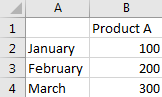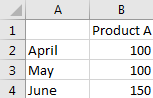a. Select a blank cell for saving the result, in this case we select E2.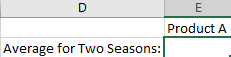b. in E2, enter the formula =AVERAGE(‘Season 1:Season 2’!B2:B4).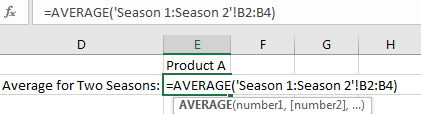Notes:

‘Season 1:Season 2’ is the worksheet range. Season 1 and Season 2 are the worksheet names. If you enter ‘sheet1:sheet3’ in this field, that means the worksheet you selected includes sheet1, sheet2 and sheet3, total three adjacent worksheets. And user need to add quotes for ‘Season 1:Season 2’.

‘B2:B4’ is the data range you want to do calculate the average.

c. Click Enter to get the result.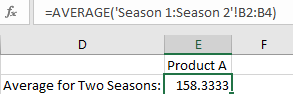## Calculate the Average for The Different Selected Ranges from Multiple Different Worksheets

First, we need to prepare two worksheets with numbers entered in the different ranges. See example below.

Worksheet1->Season 1                             Worksheet2->Season 2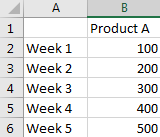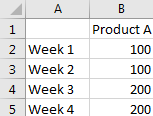a. This time, in E2, enter the formula =AVERAGE(‘Season 1′!B2:B6,’Season 2’!B2:B5). The format is ‘worksheet!data range’.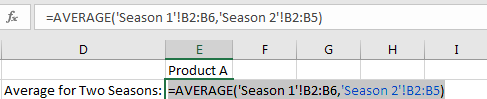b. Click Enter to get the result.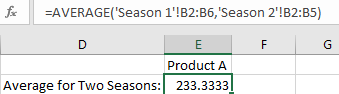### Related Functions

• Excel AVERAGE function
The Excel AVERAGE function returns the average of the numbers that you provided.The syntax of the AVERAGE function is as below:=AVERAGE (number1,[number2],…)….

Related Posts

If Cell is This Value or That Value

IF function is frequently used in Excel worksheet to return you expect “true value” or “false value” based on the result of logical test. If you want to see if a cell is A or B, and if one of ...

If Value is Greater Than A Certain Value

IF function is frequently used in Excel worksheet to return you expect “true value” or “false value” based on the logical test result. If you want to see if a value in one cell is greater than a specific value, ...

If Cell is Not Blank

IF function is frequently used in Excel worksheet to return you expect “true value” or “false value” based on the result of created logical test. If you want to see if a cell is blank or not, and leave some ...

If Cell is Blank

IF function is frequently used in Excel worksheet to return you expect “true value” or “false value” based on the result of created logical test. If you want to see if a cell is blank or not, and leave some ...

If Cell Equals Certain Text String

IF function is frequently used in Excel worksheet to return you expect “true value” or “false value” based on the result of created logical test. If you want to see if cell equals a certain text string like “Win”, you ...

If Cell Contains Either Text1 or Text2

IF function is frequently used in Excel worksheet to return “true value” or “false value” based on the logical test result. If you want to see if cell contains certain substring1 like “abc” or substring2 like “def”, and returns true ...

If Cell Contains Certain Text OR Equals Certain Text

IF cell equals certain text IF function is frequently used in Excel worksheet to return “true value” or “false value” based on the logical test result. If you want to test values to see if they equal certain text like ...

VLOOKUP From Another Sheet Not Working

In the previous post, you should know that how to fix or remove the #N/A error when using VLOOKUP formula to lookup value from another sheet. And this post will show you reasons why your VLOOKUP formula is not working ...

If Cell Begins with One of Three Supplied Characters

If you want to test values to see if they begin with some given specific characters like “x”, ”y”, or “z”, you can create a formula with COUNTIF and SUM functions to return results. EXAMPLE You can see “TRUE” or ...

Fix #N/A Error For VLOOKUP From Another Sheet

This post will show you how to fix the #N/A error why it occurs when you extract values from another sheet using VLOOKUP function in Excel 2016,2013,2010 or other Excel versions. How can you correct a #N/A error in VLOOKUP ...

Sidebar